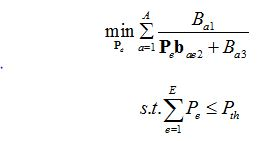# How do I represent the function of this superposition?A can be written one by one when A is smaller, but if A is larger,as A = 50, how should I describe it?
function Pe= cvx_Pe(Ba1,ba2,Ba3,Pe,A,Pth)
cvx_begin quiet
cvx_precision low
variable Pe(E,1) complex;
minimize inv_pos((Pe*bae2+Ba3)/Ba1)%I don’t know what to do here
subject to
sum(Pe)<=Pth;
cvx_end

end

try sum(inv_pos((Pe*bae2+Ba3)/Ba1)). it would be good if information about every variable’s dimension was provided.

1 Like

help inv_pos

inv_pos Reciprocal of a positive quantity.
inv_pos(X) returns 1./X if X is positive, and +Inf otherwise.
X must be real.

`````` For matrices and N-D arrays, the function is applied to each element.

Disciplined convex programming information:
inv_pos is convex and nonincreasing; therefore, when used in CVX
specifications, its argument must be concave (or affine).``````
1 Like